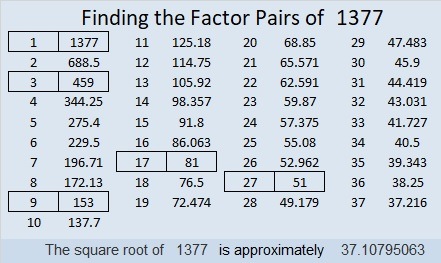Occasionally,  we hear that the number of Easter eggs that are found is one or two less than the number of eggs that were hidden. Still most of the time, all the eggs and candies do get found. You really have no trouble finding all those goodies, and the Easter Egg Hunt seems like it is over in seconds.  You can find Easter Eggs but can you find factors? Here’s an Easter Basket Find the Factors 1 – 10 Challenge Puzzle for you. I guarantee it won’t be done in seconds. Can you find all the factors? I dare you to try!Print the puzzles or type the solution in this excel file: 10 Factors 1373-1388

Now I’ll mention a few facts about the number 1377:

• 1377 is a composite number.
• Prime factorization: 1377 = 3 × 3 × 3 × 3 × 17, which can be written 1377 = 3⁴ × 17
• 1377 has at least one exponent greater than 1 in its prime factorization so √1377 can be simplified. Taking the factor pair from the factor pair table below with the largest square number factor, we get √1377 = (√81)(√17) = 9√17
• The exponents in the prime factorization are 4 and 1. Adding one to each exponent and multiplying we get (4 + 1)(1 + 1) = 5 × 2 = 10. Therefore 1377 has exactly 10 factors.
• The factors of 1377 are outlined with their factor pair partners in the graphic below.1377 is the sum of two squares:
36² + 9² = 1377

1377 is the hypotenuse of a Pythagorean triple:
648-1215-1377 which is (8-15-17) times 81
and can also be calculated from 2(36)(9), 36² – 9², 36² + 9²